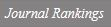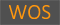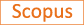Energy finite solutions of elliptic equations on Riemannian manifolds

Title
Energy finite solutions of elliptic equations on Riemannian manifolds
Authors
Kim S.W.Lee Y.H.
Ewha Authors
SCOPUS Author ID
이용하Issue Date
2008
Journal Title
Journal of the Korean Mathematical Society
ISSN
0304-9914Citation
Journal of the Korean Mathematical Society vol. 45, no. 3, pp. 807 - 819
Indexed
SCIE; SCOPUS; KCIDocument Type
Article
Abstract
We prove that for any continuous function f on the s-harmonic (1 &lt; s &lt; ∞) boundary of a complete Riemannian manifold M, there exists a solution, which is a limit of a sequence of bounded energy finite solutions in the sense of supremum norm, for a certain elliptic operator A on M whose boundary value at each s-harmonic boundary point coincides with that of f. If E1, E2,..., El are M-nonparabolic ends of M, then we also prove that there is a one to one correspondence between the set of bounded energy finite solutions for A on M and the Cartesian product of the sets of bounded energy finite solutions for A on Ei which vanish at the boundary ∂Ei for i = 1, 2,..., l. ©2008 The Korean Mathematical Society.
Appears in Collections:
사범대학 > 수학교육과 > Journal papers
Files in This Item:
There are no files associated with this item.
Export
RIS (EndNote)
XLS (Excel)
XML